## Want to keep learning?

This content is taken from the Raspberry Pi Foundation & National Centre for Computing Education's online course, How Computers Work: Demystifying Computation. Join the course to learn more.
1.10

## Raspberry Pi Foundation

Skip to 0 minutes and 1 second Now let’s have a look at why computers use binary anyway. Let’s start by thinking about the most simple use case here. Computers need to store data. Now, if computers worked in denary, then they would need to be able to store at minimum 10 different digits. So a computer would need to use some sort of electronic component that could represent 10 different states, from 0 through to 9. Now, these type of components do exist. In fact, we have components that can store almost an infinite number of states, but they become quite complicated to use in circuits. A much simpler component would be a component that just operates in only two states– on and off, for instance.

Skip to 0 minutes and 44 seconds Just such a component is called a switch. Let’s see how a switch can be used to store binary numbers. So here we have a simple circuit. You don’t need to understand what’s going on here, particularly. Just notice that up at the top, the multimeter shows a reading of 1 volt. So that’s a 1. Now, we want to be able to change the state of the circuit using a simple component. So I’m going to add in a switch.

Skip to 1 minute and 13 seconds With the switch in place, I can now control the state of the circuit by flipping the switch. When the switch is in the On position, we can see that a 1 is shown on the multimeter. When I turn the switch to the Off position, a 0 is shown on the multimeter. Now, we’ve been able to represent the values 1 and 0 using a single switch.

Skip to 1 minute and 36 seconds Now if we wanted to represent more digits, we can just add in more circuits. We can represent as large a binary number as we need. We just need one switch for every digit we want to represent. The switches used in modern computers are so cheap and so tiny, you can literally get billions of them on a single circuit board. And we’ll take a look at this in the next step.

# Why binary?

Now let’s have a look at why computers use binary anyway.

Let’s start by thinking about the most simple use case: computers need to store data. Now if a computer worked in denary, then for each digit, it would need to be able to store one of at least 10 different possibilities. At the hardware level, that would mean using some electronic component that could be in any of 10 different states, to represent the 10 digits from 0 to 9.

Such components do exist – In fact there are components that can represent an almost infinite number of states – but they are unwieldy and complicated to use in electronic circuits.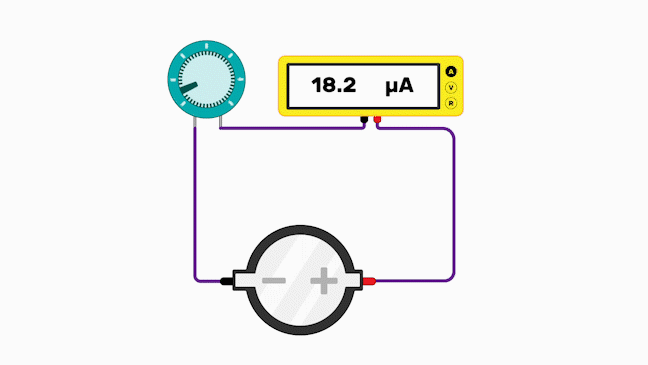A much simpler component would be one that has only two states - on and off, for instance. A component like this is called a switch.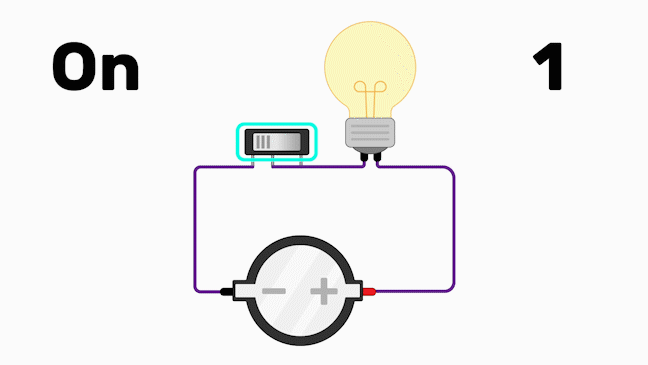Let’s have a look at how a switch can be used to store binary numbers. Shown below a simple circuit. You don’t need to understand exactly what is going on, but notice that at the top, the multimeter shows a reading of 1 volt. That is a 1.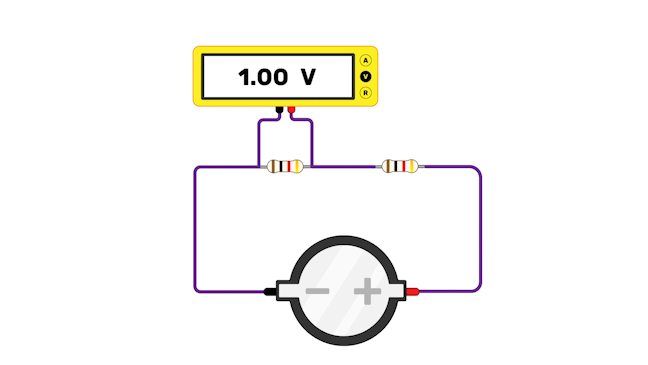We want to be able to change the state of this circuit using a simple component, so we add in a switch. With the switch in place, the circuit state can be controlled by flipping the switch.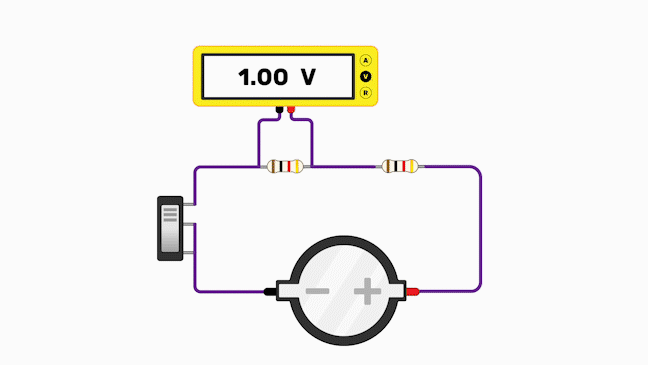When the switch is in the “on” position, a 1 is shown on multimeter. When the switch is flipped to the “off” position, a 0 is shown on the multimeter. So we have represented the numbers 1 and 0 using a simple switch.

If we want more digits, we just need to add in more circuits, and we can represent as large a binary number as we need. We just need one switch for every digit we want to represent.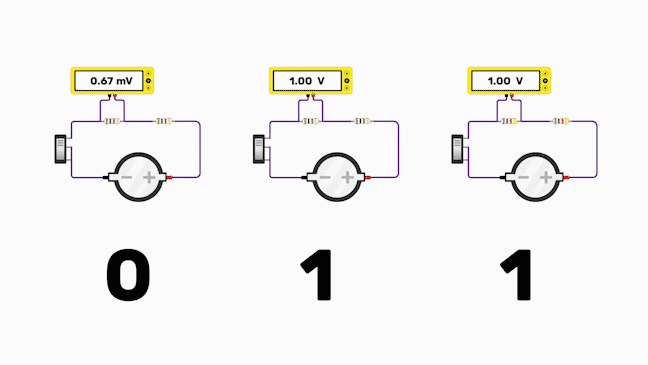The switches used in modern computers are so cheap and so small that you can literally get billions of them on a single circuit board. We’ll look at that in the next step.